# Discretizations¶

## Mathematical background¶

In mathematics, the term discretization stands for the transition from abstract, continuous, often infinite-dimensional objects to concrete, discrete, finite-dimensional counterparts. We define discretizations as tuples encompassing all necessary aspects involved in this transition. Let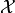be an arbitrary set,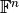be the set of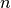-tuples where each component lies in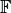. We define two mappings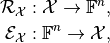which we call sampling and interpolation, respectively. Then, the discretization ofwith respect toand the above operators is defined as the tuple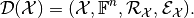The following abstract diagram visualizes a discretization: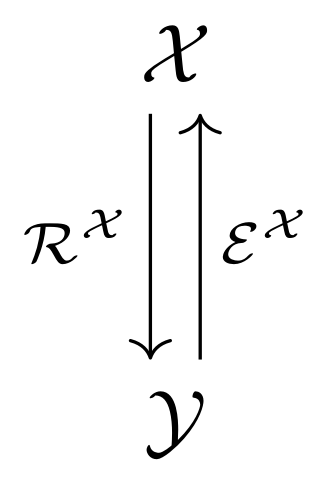TODO: write up in more detail

## Example¶

Let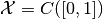be the space of real-valued continuous functions on the interval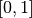, and let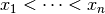be ordered sampling points in.

Restriction operator:

We define the grid collocation operator as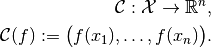The abstract object in this case is the input function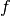, and the operator evaluates this function at the given points, resulting in a vector in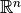.

This operator is implemented as PointCollocation.

Extension operator:

Let discrete values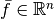be given. Consider the linear interpolation of those values at a point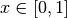: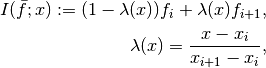whereis the index such that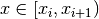.

Then we can define the linear interpolation operator as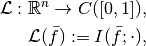where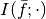stands for the function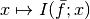.

Hence, this operator maps the finite arrayto the abstract interpolating function.

This interpolation scheme is implemented in the LinearInterpolation operator.